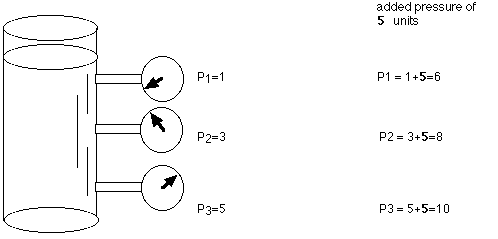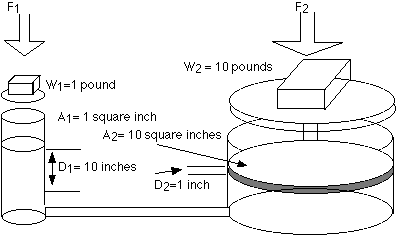+ Text Only Site
+ Non-Flash Version
+ Contact Glenn## Pascal's Principle and Hydraulics

SUBJECT: Physics
TOPIC: Hydraulics
DESCRIPTION: A set of mathematics problems dealing with hydraulics.
CONTRIBUTED BY: Carol Hodanbosi
EDITED BY: Jonathan G. Fairman - August 1996

Hydraulic systems use a incompressible fluid, such as oil or water, to transmit forces from one location to another within the fluid. Most aircraft use hydraulics in the braking systems and landing gear. Pneumatic systems use compressible fluid, such as air, in their operation. Some aircraft utilize pneumatic systems for their brakes, landing gear and movement of flaps.

Pascal's law states that when there is an increase in pressure at any point in a confined fluid, there is an equal increase at every other point in the container.

A container, as shown below, contains a fluid. There is an increase in pressure as the length of the column of liquid increases, due to the increased mass of the fluid above.

For example, in the figure below, P3 would be the highest value of the three pressure readings, because it has the highest level of fluid above it.If the above container had an increase in overall pressure, that same added pressure would affect each of the gauges (and the liquid throughout) the same. For example P1, P2, P3 were originally 1, 3, 5 units of pressure, and 5 units of pressure were added to the system, the new readings would be 6, 8, and 10.

Applied to a more complex system below, such as a hydraulic car lift, Pascal's law allows forces to be multiplied. The cylinder on the left shows a cross-section area of 1 square inch, while the cylinder on the right shows a cross-section area of 10 square inches. The cylinder on the left has a weight (force) on 1 pound acting downward on the piston, which lowers the fluid 10 inches. As a result of this force, the piston on the right lifts a 10 pound weight a distance of 1 inch.

The 1 pound load on the 1 square inch area causes an increase in pressure on the fluid in the system. This pressure is distributed equally throughout and acts on every square inch of the 10 square inch area of the large piston. As a result, the larger piston lifts up a 10 pound weight. The larger the cross-section area of the second piston, the larger the mechanical advantage, and the more weight it lifts.The formulas that relate to this are shown below:

P1 = P2 (since the pressures are equal throughout).

Since pressure equals force per unit area, then it follows that

F1/A1 = F2/A2

It can be shown by substitution that the values shown above are correct,

1 pound / 1 square inches = 10 pounds / 10 square inches

Because the volume of fluid pushed down on the left side equals the volume of fluid that is lifted up on the right side, the following formula is also true.

V1 = V2

by substitution,

A1 D1 = A2 D2
• A = cross sectional area
• D = the distance moved

or

A1/A2= D2/D1

This system can be thought of as a simple machine (lever), since force is multiplied.The mechanical advantage can be found by rearranging terms in the above equation to

Mechanical Advantage(IMA) = D1/D2 = A2/A1

For the sample problem above, the IMA would be 10:1 (10 inches/ 1 inch or 10 square inches / 1 square inch).

Given these simple formulas, try to answer the questions below.

Exercises:

1. A hydraulic press has an input cylinder 1 inch in diameter and an output cylinder 6 inches in diameter.
1. Assuming 100% efficiency, find the force exerted by the output piston when a force of 10 pounds is applied to the input piston.

2. If the input piston is moved through 4 inches, how far is the output piston moved?

2. A hydraulic system is said to have a mechanical advantage of 40. Mechanical advantage (MA) is FR (output) / FE (input). If the input piston, with a 12 inch radius, has a force of 65 pounds pushing downward a distance of 20 inches, find

1. the volume of fluid that has been displaced

2. the upward force on the output piston

3. the radius of the output piston

4. the distance the output piston moves

3. What pressure does a 130 pound woman exert on the floor when she balances on one of her heels? Her heels have an average radius of 0.5 inch.

4. A car has a weight of 2500 pounds and rests on four tires, each having a surface area of contact with the ground of 14 square inches. What is the pressure the ground experiences beneath the tires that is due to the car?

Extension :
5. The input and output pistons of a hydraulic jack are respectively 1 cm and 4 cm in diameter. A lever with a mechanical advantage of 6 is used to apply force to the input piston. How much mass can the jack lift if a force of 180 N is applied to the lever and efficiency is 80%?+ Inspector General Hotline + Equal Employment Opportunity Data Posted Pursuant to the No Fear Act + Budgets, Strategic Plans and Accountability Reports + Freedom of Information Act + The President's Management Agenda + NASA Privacy Statement, Disclaimer, and Accessibility CertificationEditor: Tom Benson NASA Official: Tom Benson Last Updated: May 13 2021 + Contact Glenn